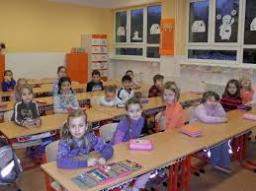# Children 6303

A total of 56 children went on a trip to the pupils of the two fourth grades. That 4A was four pupils more than 4. B How many pupils from each class went on a trip?

a =  30
b =  26

### Step-by-step explanation:

a+b=56
a = 4+b

a+b = 56
a-b = 4

Row 2 - Row 1 → Row 2
a+b = 56
-2b = -52

b = -52/-2 = 26
a = 56-b = 56-26 = 30

a = 30
b = 26

Our linear equations calculator calculates it.Did you find an error or inaccuracy? Feel free to write us. Thank you!

Tips for related online calculators
Do you have a system of equations and looking for calculator system of linear equations?